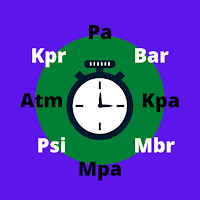Pressure Converter - Measure Pressure# Pressure Converter - Measure Pressure

## PH Solution

A wonderful app for converting between the different units of pressure
Free

### Screenshots for App

Mobile
Pressure(symbol: 'P' or 'p') is the force applied perpendicular to the surface of an object per unit area over which that force is distributed.

The pressure is distributed to solid boundaries or across arbitrary sections of fluid normal to these boundaries or sections at every point. It is a fundamental parameter in thermodynamics, and it is conjugate to volume.

Mathematically: P= F/A
where: p is the pressure,
F is the magnitude of the normal force,
A is the area of the surface on contact.

The different types of pressure are differentiated only by the reference pressure.
Absolute pressure
Atmospheric pressure
Differential pressure
Overpressure (gauge pressure)

The SI unit for pressure is the pascal (Pa), equal to one newton per square meter (N/m2, or kg·m−1·s−2). This name for the unit was added in 1971; before that, pressure in SI was expressed simply in newtons per square meter.

Other units of pressure, such as pounds per square inch (Ibf/in2) and bar, are also in common use. The CGS unit of pressure is the barye (Ba), equal to 1 dyn·cm−2, or 0.1 Pa. The pressure is sometimes expressed in grams-force or kilograms-force per square centimeter (g/cm2 or kg/cm2) and the like without properly identifying the force units.

FORMULA FOR CONVERTING KILO PASCAL TO PASCAL:~

One pascal is the pressure exerted by a force of magnitude one newton perpendicularly upon an area of one square meter.

1 Pascal = 1 Newton / m²

Kilopascal (kPa), one thousand times the unit of pressure and stress in the meter-kilogram-second system (the International System of Units [SI]).

1 Kilo Pascal = 1000 Pascal

FORMULA FOR CONVERTING MEGA PASCAL TO PASCAL:~

The megapascal is an x1000000 multiple of the Pascal unit which is the SI unit for pressure. 1 megapascal equals 1,000,000 pascals.
Primarily used for higher range pressure measurement due to its larger value (e.g. 1 MPa = 10 bar), the MPa is mainly used to describe the pressure ranges and ratings of hydraulic systems.

1 Mega Pascal = 10⁶ Pascal

FORMULA FOR CONVERTING BAR TO PASCAL:~

The bar is a metric unit of pressure, but not part of the International System of Units (SI). It is defined as exactly equal to 100,000 Pa (100 kPa), or slightly less than the current average pressure at sea level (approximately 1.013 bar).

1 Bar = 100,000 Pascal

FORMULA FOR CONVERTING MILLI BAR TO PASCAL:~

Millibar(mbar), unit of air pressure in the metric system, commonly used in meteorology, equal to 100 pascals, 1,000 dynes per square cm (about 0.0145 pounds per square inch), or slightly less than one-thousandth of a standard atmosphere.

1 Millibar = 100 Pascal

KEY FEATURES OF THE PRESSURE CONVERTER APP:~

I)The Pressure Converter App converts the major 8 units of pressure to Pascal, Kilo Pascal, MegaPascal,Bar, Millibar, Atmospheric pressure(atm), Pound force per square inch (psi), and Dyne per square centimeter.

II)The Pressure converter app not only helps in scientific conversions without any mathematical derivation.It also helps to measure air pressure.

III)Just enter the value of pressure in any of these units and the other 7 units will get converted automatically in just a few seconds as an advantage.

IV)The Pressure Converter App determines the value of pressure in precise decimal points that is no matter of round figuring is considered.

V)The app is completely free to use and no personal information is required.

The Area Converter App is developed by PH SOLUTION.